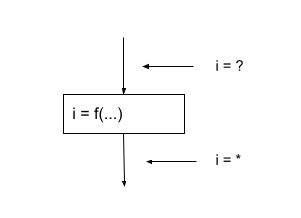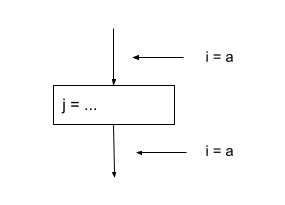×

Search anything:

# Constant Propagation in Compiler Design

#### Compiler Design Software EngineeringGet this book -> Problems on Array: For Interviews and Competitive Programming

• Introduction
• Example Uses
• Global Analysis
• Algorithm for Analysis
• Overview

Pre-requisites:

# Introduction

In this article we will cover the basis of constant propagation, an optimisation technique used on local code. Constant propagation is the process of replacing constant values of variables in an expression. We are replacing a value which is a known constant simply with that value instead of assigning a calculation for the constant. This reduces operations and increases efficiency.

# Example Uses

``````Pi = 22/7

Vs

Pi = 3.14
``````

In this case, the first assignment of Pi will be less efficient than the second one due to the fact that an operation is involved. Each time that Pi is used in the program, the compiler has to look up the value, compute the division and assign it to Pi before it is used. This is inefficient has an expensive operation is potentially being used multiple times therefore, it is more efficient to assign the value of 3.14 directly to Pi so that each access requires less time and by extension, reducing the runnig time of our code.

``````i = 25
j = 20 - i/2
k = j * (30 / i + 2) - i

Vs

i = 25
j = 20 - 25/2
k = j * (30/25+2) - 25
``````

Here we have two pieces of code which use constants to calculate values. The second version is faster than the first version due to increased propagation. Firstly 25 is assigned to i, this means that when the compiler reaches j, it will have to go back to the i expression, find the value of i in which 25 is assigned to it again, only then can it go and execute the second expression. When it reaches the third expression, the compiler will have to evaluate the first and second expression once more in order to be able to calcluate the third. This means that the variable i will have to be propagated three times which is very detrimental to performance.

The second version increases efficiency since the compiler does not have to recalculate the values before the one it is computing in order to find the value of its current expression. It means that complexity is reduced and operations are performed more efficiently, reducing running time.

Constant propagation does rely on the individual compiler as they peform it in different ways, we will explain these techniques below.

# Global Analysis

Constant propagation relies on global analysis in order to effectively be carried out. This is because we need to know how data flows across the program in order to know where it is okay to carry out propagation.

To execute constant propagation optimisation we need to know several things:

• A property which we want to optimise at a particular point in the program (P)
• To prove P at any point, we need to analyse the entire function it is contained in
• For example, if P needs to be true then we should know:
• P is definitely true or,
• We don't know if P is true

Global constant propagation can be performed at any point in which a property P stands.

To explain this further, let's say that we need to calculate property P for a variable i.

We can associate values with i at various program points.

Value Meaning
# Statement is never executed
c i = constant c
* Unknown whether i is a constant

Take for example this program:

``````i = 42
j = x * z
k = j + i
``````

This can be optimised using constant propagation to:

``````i = 42
j = x * z
k = j + 42
``````

And further once more with dead code elimination, i can be eliminated

``````j = x * z
k = j = 42
``````

Using our table of values we can plot a control-flow graph for this program in the following way:From here it is fairly simple, we can inspect i = ? with a statement using i. If i is constant, replace that instance of i with the constant. The challenge comes with having to calculate the properties of i = ?.

To do this, we can transfer information from one statement to the next. For everysing statement (S), we compute the information about i before and after s. Using this logic, we can deduce two expressions:

Cin = value of i before statement s
Cout = value of i after statement s

We should define a transfer function which transfers information from one statement to the next. This therefore means that the statement s will have predecessing statements p1,...,pn.

There are multiple rules that we need to explicate in order to do this:

## Rule 1If Cout(x, Pi) = * for all i, then Cin(x, s) = *

## Rule 2If Cout(x, Pi) = c and Cout(x, pj) = d and d != c then Cin(x, s) = *

## Rule 3If Cout(x, Pi) = c or # for every i, then Cin(x, s) = c

## Rule 4If Cout(x, Pi) = # for every i, then Cin(x, s) = #

So far, our rules have related to the out of one statement to the in of its successor, there are also rules regarding the in and out of the same statement.

## Rule 5Cout(x, s) = # if Cin(x, s) = #

## Rule 6Cout(x, x = c) = c if c is a constant

## Rule 7Cout(x, x = f(...)) = *

## Rule 8Cout(x, y = ...) = cin(x, y = ...) if x != y

# Algorithm for Analysis

Using our rules which we have defined above, we can create an algorithm to apply them.

• For every input s to the function, Cin(x, s) = *
• Cin(x, s) = Cout(x, s) = # all other places
• Repeat until all statements satisfy rules 1 - 8
• Choose statement which doesn't satisfy rules 1 - 8 and updates it using the approptiate rule

# Overview

In this article at OpenGenus, we have discussed the concept of constant propagation and how it is used. We have gone over the principles of how it is executed including the use of global analysis and its rules. After reading this article, you will have a good understanding of global constant propagation, as well as compiler principles such as global code analysis.